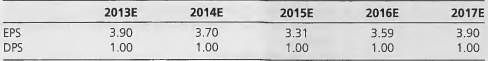### Create an Account

Home / Questions / Residual Earnings Valuation and Target Prices Medium The following forecasts of earnings p...

# Residual Earnings Valuation and Target Prices Medium The following forecasts of earnings per share E PS and dividend per share DPS were made at the end of 2012 for a firm with a book value per

Residual Earnings Valuation and Target Prices (Medium) The following forecasts of earnings per share (E PS) and dividend per share (DPS) were made at the end of 2012 for a firm with a book value per share of \$22.00:The firm has an equity cost of capital of 12 percent per annum.

a. Calculate the residual earnings that are forec ast for each year, 2013 to 2017.

b. What is the per-share value of the equity at the end of 2012 based on the residual income valuation model?

c. What is the forecasted per-share value of the equity at the end of the year 2017? d. What is the expected premium in 2017?

May 26 2020 View more View LessSubscribe To Get Solution